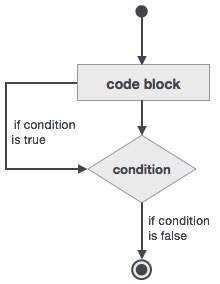# Fortran - do while Loop Construct

It repeats a statement or a group of statements while a given condition is true. It tests the condition before executing the loop body.

## Syntax

```do while (logical expr)
statements
end do
```

## Flow Diagram## Example

```program factorial
implicit none

! define variables
integer :: nfact = 1
integer :: n = 1

! compute factorials
do while (n <= 10)
nfact = nfact * n
n = n + 1
print*,  n, " ", nfact
end do
end program factorial
```

When the above code is compiled and executed, it produces the following result −

```2             1
3             2
4             6
5            24
6           120
7           720
8          5040
9         40320
10        362880
11       3628800
```
fortran_loops.htm